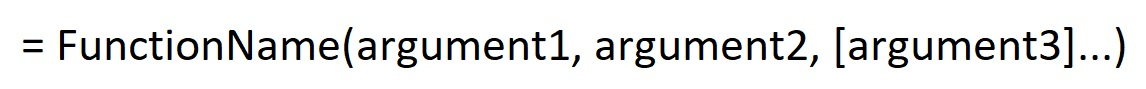# Excel Functions: Function BasicsFunctions are predetermined formulas that perform specific computations. For example, say you want to find the total of the range A1-A30. Instead of manually entering the formula = A1+A2+A3+….+A30, you can use the sum function to perform the same task much faster.

Functions have predefined names. For example, the sum function can be used to add a group of numbers; the average function can be used to find the average of a group of numbers, etc. Functions are bound by syntax and logic similar to all formulas.

### Syntax

All Excel functions appear in the following form:2. The name of the function follows the equal sign. For example, to use the sum function, type =sum .
3. The contents of the function are enclosed in a pair of parentheses ( ) following the name of the function.
4. Inside the pair of parentheses are the arguments of the function. Arguments, or sometimes called variables, are the pieces of information that must be provided to execute the function and yield results. For example, for the sum function, the arguments are the numbers to be added up.
5. Each argument must be separated by a comma.
6. Some arguments may be optional (i.e., they are defaulted by Excel or they do not have an effect even if they are omitted). These arguments are denoted inside a pair of square brackets. For example, the sum function can handle up to 255 arguments. If only 2 arguments are entered (e.g., =sum(10,20)), arguments 3-255 are not needed (or defaulted as zero).
7. Cell references can be used for functions. (For more details about cell references, see Cell References.)

### Logic

Below is the order of Excel function execution:

Order of OperationOperator
1stFunctions inside functions
2nd( )
3rd^
4th* /
5th+ –
6thThe function itself
1. Multiplication and division must be performed before addition and subtraction.

Example: For the formula = sum(1+2*3,4), the multiplication is calculated first. The first argument is 7 (1 + 6), and the function returns the result of 11 (7 + 4).

1. Exponents (powers) are calculated before multiplication and division.

Example:For the formula = sum(1, 2^3 * 4), the exponent is calculated first, followed by the multiplication. The second argument is 32 (2^3 * 4), and the function returns the result of 33 (1 + 32).

1. Calculations inside parentheses are executed before mathematical operators.

Example: For the formula =sum((1 + 2) ^ 3 – 4,5), the addition within the innermost pair of parentheses is done first, followed by the exponent and the subtraction. The first argument is 23 (3^3 – 4), and the function returns the result of 28 (23 + 5).

1. Functions inside functions are calculated first.

Example: For the formula = sum(sum(1,2,3)*4, 5), the innermost function is run first. The first argument is 24 (6 * 4), and the outermost function returns the result of 29 (24 + 5).

### Learning Excel Data Analytics with Gleim

At Gleim, we know learning data analytics is vital for future accounting professionals. That is why we are offering these series of data analytics blogs and continually updating all of our CPA, CMA, and CIA Review materials with the necessary information you need to pass your exams.

We’ll continue our weekly blog series. Check back regularly for exam news and study tips!

Python Lessons
Python Basics
Conditional Statements
Loops
Functions and Modules
Numerical Python (NumPy)
Pandas Basics
Pandas Data Capture and Cleansing
Merging and Grouping
Manipulating Text and Datetime Data
Visualization
Web Scraping
Errors and Exceptions